Home

# Multipliers on Vector Valued Bergman Spaces

## Abstract

Let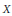$X$ be a complex Banach space and let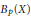${{B}_{p}}\left( X \right)$ denote the vector-valued Bergman space on the unit disc for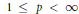$1\,\le \,p\,<\,\infty$ . A sequence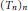${{\left( {{T}_{n}} \right)}_{n}}$ of bounded operators between two Banach spaces$X$ and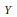$Y$ defines a multiplier between${{B}_{p}}\left( X \right)$ and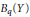${{B}_{q}}\left( Y \right)$ (resp.${{B}_{p}}\left( X \right)$ and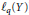${{l}_{q}}\left( Y \right)$ ) if for any function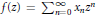$f\left( z \right)\,=\,\sum _{n=0}^{\infty }\,{{x}_{n}}{{z}^{n}}$ in${{B}_{p}}\left( X \right)$ we have that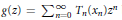$g\left( z \right)\,=\,\sum _{n=0}^{\infty }\,{{T}_{n}}\left( {{x}_{n}} \right){{z}^{n}}$ belongs to${{B}_{q}}\left( Y \right)$ (resp.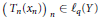${{\left( {{T}_{n}}\left( {{x}_{n}} \right) \right)}_{n}}\,\in \,{{\ell }_{q}}\left( Y \right)$ ). Several results on these multipliers are obtained, some of them depending upon the Fourier or Rademacher type of the spaces$X$ and$Y$ . New properties defined by the vector-valued version of certain inequalities for Taylor coefficients of functions in${{B}_{p}}\left( X \right)$ are introduced.

• # Send article to Kindle

Note you can select to send to either the @free.kindle.com or @kindle.com variations. ‘@free.kindle.com’ emails are free but can only be sent to your device when it is connected to wi-fi. ‘@kindle.com’ emails can be delivered even when you are not connected to wi-fi, but note that service fees apply.

Find out more about the Kindle Personal Document Service.

Multipliers on Vector Valued Bergman Spaces
Available formats
×

# Send article to Dropbox

To send this article to your Dropbox account, please select one or more formats and confirm that you agree to abide by our usage policies. If this is the first time you use this feature, you will be asked to authorise Cambridge Core to connect with your <service> account. Find out more about sending content to Dropbox.

Multipliers on Vector Valued Bergman Spaces
Available formats
×

# Send article to Google Drive

To send this article to your Google Drive account, please select one or more formats and confirm that you agree to abide by our usage policies. If this is the first time you use this feature, you will be asked to authorise Cambridge Core to connect with your <service> account. Find out more about sending content to Google Drive.

Multipliers on Vector Valued Bergman Spaces
Available formats
×

## References

Hide All
MathJax
MathJax is a JavaScript display engine for mathematics. For more information see http://www.mathjax.org.

# Multipliers on Vector Valued Bergman Spaces

## Metrics

### Full text viewsFull text views reflects the number of PDF downloads, PDFs sent to Google Drive, Dropbox and Kindle and HTML full text views.

Total number of HTML views: 0
Total number of PDF views: 0 *Loading metrics...

### Abstract viewsAbstract views reflect the number of visits to the article landing page.

Total abstract views: 0 *Loading metrics...

* Views captured on Cambridge Core between <date>. This data will be updated every 24 hours.

Usage data cannot currently be displayed.